Courses

# Test: Area Of Combination Of Figures

## 10 Questions MCQ Test Mathematics (Maths) Class 10 | Test: Area Of Combination Of Figures

Description
This mock test of Test: Area Of Combination Of Figures for Class 10 helps you for every Class 10 entrance exam. This contains 10 Multiple Choice Questions for Class 10 Test: Area Of Combination Of Figures (mcq) to study with solutions a complete question bank. The solved questions answers in this Test: Area Of Combination Of Figures quiz give you a good mix of easy questions and tough questions. Class 10 students definitely take this Test: Area Of Combination Of Figures exercise for a better result in the exam. You can find other Test: Area Of Combination Of Figures extra questions, long questions & short questions for Class 10 on EduRev as well by searching above.
QUESTION: 1

### If a square is inscribed in a circle, then the ratio of their areas is​

Solution: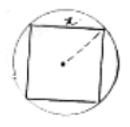Let side of square be x cms inscribed in a circle.

Radius of circle (r) = 1/2(diagonal of square)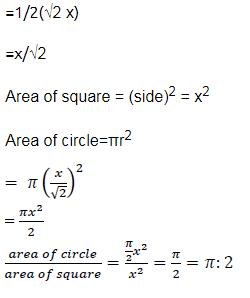QUESTION: 2

### In the given figure, O is the centre of the circle, and AC is its diameter. Another circle with AB as diameter is drawn. If AC = 54 cm and BC = 10 cm, the area of shaded portion will be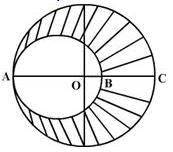​

Solution:

AC=54cm, Radius=AO=54/2=27cm
Area of the bigger circle= πR2= 22/7 * 27 * 27=2291 (approx)
Diameter of the smaller circle=54-10=44
Radius=44/2=22
Area of the smaller circle= πr2=22/7 * 22 * 22=1521 (approx)
Area of the shaded region=Area of the bigger circle-Area of the smaller circle
= 2291-1521=770 cm2

QUESTION: 3

### The perimeter of the given figure is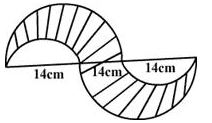Solution:

Perimeter is the boundary of the figure
So we have two semicircles of radius 14cm which makes one complete circle. Also there are two semicircles of radius 7 cm which makes one circle.
So perimeter= 2πR+2πr=2(R+r)=2*22/7(14+7)=22*6=132cm

QUESTION: 4

If PQRS is a square of side 14 cm and PAQ and SAR are semi-circles, then the area of the shaded region is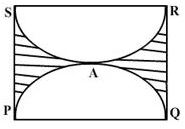Solution:
QUESTION: 5

What is the area of the square inscribed in a circle with a circumference of 18.84 cm?​

Solution: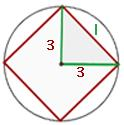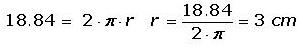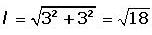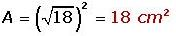QUESTION: 6

A park is in the form of a rectangle 120m by 90 m. At the centre of the park there is a circular lawn as shown in the figure. If the area of the park excluding the lawn is 2950 m2, then the radius of the circular lawn will be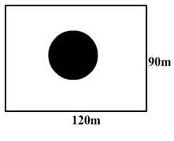Solution:

ANSWER :- b

Solution :- Area of rec. park=(120×90)m²=10800m²

Area of rec. park excluding lawn=2950m²

Area of circular lawn=(10800 - 2950)m²

=7850m²

Let the radius be = r

7850/3.14 = 3.14r²/3.14

→2500 = r²

→√2500 = √r²

→50 = r

→r = 50

Radius = 50m

QUESTION: 7

Calculate the area of the designed region in the given figure common between the two quadrants of circles of radius 8 cm each.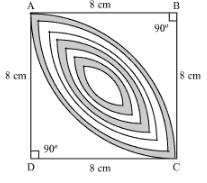Solution:
QUESTION: 8

From a circular sheet of paper with a radius 20 cm, four circles of radius 5 cm each are cut out. What is the ratio of the uncut to the cut portion?​

Solution:

Four circles of radius 5 cm are cut out.
Area cut = 4*(π52) = 100π
Total area of circular sheet = π(20)2 = 400π
Area uncut = 300 π
The ratio of uncut to cut portion is 3 : 1

QUESTION: 9

A momento is made as shown in the figure. Its base, shade is to be silver plated from the front at the rate of rs 20 per cm2. What is the total cost of silver plating?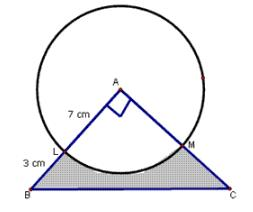Solution:
QUESTION: 10

In given figure, a circle of radius 7.5cm is inscribed in a square, the remaining area of the square is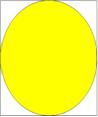Solution: